Riemann was one of the finest pure mathematicians of his century.  ...within his mind burned the desire to understand the nature of the physical world...Anticipating relativity and modern cosmology, Riemann understood that in order to grasp the meaning of the physical world, one had to develop a deep understanding of...geometry.Amir D. Aczel [circa 1953- ]    -God's Equation, 1999 The general theory of relativity splendidly justified his (Riemann's) work.In the mathematical apparatus developed from Riemann's address, Einstein found the frame to fit his physical ideas, his cosmology, and cosmogony: and the spirit of Riemann's address was just what physics needed: the metric structure determined by data.Hans Freudenthal [1905-1990]   -Dictionary of Scientific Biography, 1970-90
 I've often wondered how Einstein could...make such a simple assumption... the universe is so simple that we can analyze it in a one-dimensional differential equation--everything a function of time...Einstein...was awfully close to the truth--that's the way the universe looks. James Peebles [1935- ]    -Origins: The Lives and Worlds of          Modern Cosmologists, 1990,                by A. Lightman and R. Brawer Researchers have hypothesized that Einstein extended his quest to the entire universe because of an idea of Ernst Mach. ...Mach's law of inertia.  ...says that...inertia...is an effect...due to...all other mass in the universe. Amir D. Aczel [circa 1953- ]    -God's Equation, 1999; and Max Born [1882-1970]    -Einstein's Theory of Relativity, 1965
 Simplistic, Unimetric, two-dimensional, non-inflationary, alternative, heuristic Overview of  Reality: Seminal motion manifests as an unending, elliptical pulse, which is described by Brunardot Ellipses forming quaquametrically about an Infinity Line.  Said ellipses are defined by the Brunardot Series. Brunardot Ellipses define the shape of Reality, a singularity, as spherical; and, the shape of the Universe as elliptical. Brunardot Ellipses demonstrate, as the Brunardot Series reveals, that the Fibonacci series is incomplete because two of its most salient terms are not included . . . the very terms which establish its enigmatic character. Brunardot Harmonic Ellipses define the internal structure of mass and Light and the source and destination of their unending dissipation . . . and entanglement. The compression of Pulsoids, which are Brunardot Ellipsoids, and  its counter reaction manifests as gravitational "attraction" and Cosmic Inertia. Pulsoids are prototypical mass and Light; the source of  most "dark" matter and "dark" energy.  Pulsoids are analogous to Einstein's fields and the "fabric of space."  The Brunardot  Series is unique in that it defines certain classes of ellipses; all of which determine the Conceptual Unit . . ."One," 1.  The Conceptual Unit is referred to as "the Proof of One."The Brunardot Series, additionally, locks together the Golden Ratio, Phi, F; Pi, p, the ratio of the circle; and, completes the Fibonacci series as the second sequence of the Brunardot Series.
 Unimetry describes the Natural creation of mathematicsthat begins with Brunardot Ellipses,which are two-dimensional, heuristic,manifestations of a seminal pulse.
 Unimetric Structural Parts of the ellipse

 Ellipses are defined by the Brunardot Theorem: c2 = 2v2 - s2. c = major chord, v = vector, s = soliton.

 The Pulser of an ellipse defines every ellipse.Said Pulser is the perigee, p; and vector, v.The vector always equals the sum of the perigee and the soliton, s: v = p + s. The cardinal value of any two terms, or structural parts, of any ellipse, defines that ellipse.

 The Brunardot Series consists of unending sequences, each with unending terms, that have any Natural integer for the first term of each sequence and,the third term is the square of the first term; and,as with successive terms,the third term is the sum of the preceding two terms.

 The ratios of the terms of any Brunardot Series Sequence converge at Phi, F, the Golden Ratio, as the terms increase, when any term is divided by the preceding term. Phi, F, the Golden Ratio equals one plus the square root of 5, all divided by 2, or, 1.618...,

 Sequences of the Brunardot Series
 0 0 0 0 0 0 0 0 0 0 0 ... Infinity 1 0 1 1 2 3 5 8 13 21 34 ... Reality 2 2 4 6 10 16 26 42 68 110 178 ... Universe 3 6 9 15 24 39 63 102 165 267 432 ... "  " 4 12 16 28 44 72 116 188 304 492 796 ... "  " 5 20 25 45 70 115 185 300 485 785 1270 ... "  " 6 30 36 66 102 168 270 438 708 1146 1854 ... "  " 7 42 49 91 140 231 371 602 973 1575 2548 ... "  " 8 56 64 120 184 304 488 792 1280 2072 3352 ... "  " ... ... ... ... ... ... ... ... ... ... ... ... ...

 The Golden Sequence is an interesting sequence in the manner of a Brunardot Series Sequence beginning with Phi, F.  Note that the coefficients, of Phi, F, in the second form of the Golden Sequence, match the "completed" or "revised" Fibonacci series, which generates the sphere of Reality.
 F 1 F2 F2 +1 2F2 +1 3F2 +2 5F2 +3 8F2 +5 13F2 +8 21F2 +13 34F2 +21 Golden Sequence
 F2 = F + 1; therefore, rewriting the above, yields the following: F 1 F +1 F +2 2F +3 3F +5 5F +8 8F +13 13F +21 21F +34 34F +55 Golden Sequence The coefficients of F, above, yield, below, the "revised" Fibonacci Series with the added first two terms, which is the second Brunardot Series Sequence: 1 0 1 1 2 3 5 8 13 21 34 55 The above "revised" Fibonacci Series' first four terms, as perigee, soliton, vector, and apogee, generate a Brunardot Ellipse in the form of a circle with a radius of the  Conceptual Unit, 1, (r = 2p - 1).  This circle, as the seminal pulse, describes the locus of the sphere of Reality.

 Within the Brunardot Series; and the related Tinicir arrays, and their elliptic, circular, and generated three- and four-dimensional manifestations; are found all the fundamental concepts of all the fundamental phenomena of Reality: mathematics and physics . . . science, theology, and philosophy.

 Tinicir is an acronym for Tangent, Infinite, Integer Circles. Tinicirs have curvatures that are Natural Integers; and, Tinicirs are similar to Brunardot Ellipses because they are generated from unending sequences with unending terms that are Natural Integers.

 Click Any Image for a Larger View A Tini Circle Group contains circles wherein every circle is tangent to its adjacent circles; and, every curvature (the reciprocal of a circle's radius, 1/r) is an integer. There is a circle, with a decreasing integer radius, that is tangent to all adjacent circles that continues to the infinitesimal, for every open space between every tangent circle, and within, every tangent circle; that is:
 Within any group of three mutually tangent integer circles that are within any circle, there exists no space, to the infinitesimal, that does not contain an integer radius circle that is tangent to all of its adjacent group of three mutually tangent circles. The formula, for four mutually tangent circles (where a, b, c, and d represent the circles' curvature), is: a2 + b2 + c2 + d2 = (a + b + c + d)2  / 2 The curvature is negative or positive depending upon whether the curvature is concave or convex.
 Every integer radius belongs to an array that contains endless sequences and terms; each array is defined by two, and occasionally only one, integer; these defining integers are referred to as: Tini Cirts.  Tini Cirts are an acronym for Tangent, Infinite, Integer, Circle Terms. There are at least three Tini Cirts that begin with the integer One, 1, for every other Natural Integer, above Zero, there are more Tini Cirts.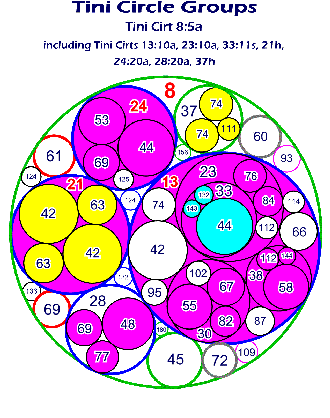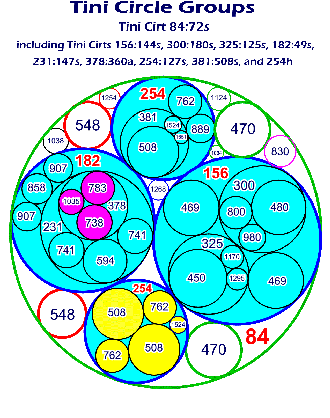Click Any Image for a Larger View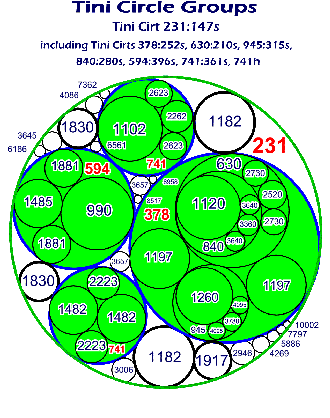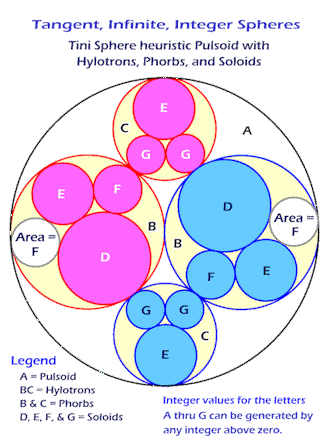The heuristic Pulsoid, above lower right, has twelve tangent inner spheres, each of which, represents a solitonic pulse. These solitonic pulses exchange energy with their adjacent pulses, which balances and sustains each soliton.  This exchange of energy between the solitons is referred to as a: Solitonic Bond. Solitonic Bonds are the fundamental phenomenon that creates the bonds of sub-atomic particles, atoms, and molecules. The internal energy is provided from the chain of Tini Circle Spheres generated from the infinitesimal.

 Brunardot Ellipses are defined by a Pulser, which is:the first and third terms of any Brunardot Series; thus, the Brunardot Elliptic Theorem is: v = p2. The cardinal value of either term of the Pulser of a Brunardot Ellipse, or any other single, structural part, defines a unique Brunardot Ellipse.

 The six most salient structural parts of Brunardot Ellipses are: perigee, p; soliton, s; vector, v; diagonal, d; radius, r; and, force, f. Other structural parts are: apogee, a; and, major chord, c.

 The perigee can be any Natural Integer; the soliton is an even integer; the vector is a square, as is the radius in the higher ellipses. The diagonal is unusually salient; the diagonal is a Natural Prime number, which demonstrates that said Natural Prime numbers are uniformly distributed, as the diagonal can be mapped to the perigee; which can be any Natural Integer. The distribution of conventional prime numbers appears random because they are the result of three combined sets of Natural Prime numbers.

 Some Important Terms, Factors, and Ratios of Brunardot Ellipses are: CU = Conceptual Unit, HF = Harmonic Factor, and K = Unimetric Factor.

 Primary Corollaries of the Brunardot Elliptic Theorem are: 2p - r = d - 2s = 2d - r2 = r2 -  4s = p - K = CU = 1,2s + 1 = (r2 + 1) / 2 = 2v - r = d, 2p - 1 = v - s2 / v = SqRt(2d - 1) = r, SqRt(rv) = pSqRt(r) = HFp = f,  (r2 - 1) / 4 = p2 - p = s,a = 2p2 - p,  v = (a + p) / 2.

 The only universal constant is the Conceptual Unit, One, 1; the speed of Light is inversely proportional to the local value of Light, L, or 2s.

 There are many classes of Brunardot Ellipses.Four such classes are:Seminal Ellipses, Natural Ellipses,Conceptual Ellipses, and Harmonic Ellipses.

 Seminal Ellipses are the simplest, or first order, of Brunardot Ellipses, which havethe soliton, s, and the diagonal, d,as Natural integers.

 Natural Ellipses are Seminal Ellipseswhich have the perigee, p; and the vector, v;  as Natural Integers.

 Conceptual Ellipses are Natural Ellipses,  which have the force, f, as a Natural integer; and, the radius, r, as a square. Every Natural Integer generates a unique Conceptual Ellipse.

 Harmonic Ellipses are Conceptual Ellipses that are generated by every Conceptual Ellipse in unending iterations, of four cycles. Each Harmonic Ellipse is defined by its cycle within an iteration, i, and its generating integer.

 All Brunardot Ellipses, in accordance with the subsequent terms of each Brunardot Series Sequence, converge to a Unimetric Ellipse. A Unimetric Ellipse is defined by a Pulser that is any two adjacent terms, after the first term, of a Brunardot Series Sequence.

 The Unimetric Ellipse is an ellipse, which is similar to an ellipse with a Pulser of: 1/F and F. F, Phi, the Golden Ratio is: (The Square Root of Five, 5, plus One, 1) divided by Two, 2. The soliton and radius of a Unimetric Ellipse are: each equal to a Conceptual Unit, CU, One, 1; also, for all Unimetric Ellipses: s / p = v / s = a / v = Phi, F.

 Unimetric Ellipsoids, when compressed from every direction, evolve to Tinisoids, which are analogous to Pulsoids. Tinisoids are heuristically described, two-dimensionally, by Tinicirs.

 There are four forms of Tinicirs. Tinicirs are described by two terms that establish the largest first two tangent circles.  All following smaller tangent circles are of three types which are determined

 Before time, there was a soundless, infinitesimal pulse. Its locus is Infinity. Its shape is spherical . . . a special ellipse.  Because of its congruity, it is a singularity. This spherical pulse is referred to as . . . Reality. And; with limitless time; subsequent, elliptic pulses; and, their sequential and cyclic evolution: converge, coalesce, compress, and dissipate; this simple complexity becomes the sustaining, endless, cyclical Universe.

 The Seminal Pulse, Reality, is defined by the first three terms, of the second sequence, of the Brunardot Series.  The second sequence is the "revised" Fibonacci Series.

 The second sequence of  the Brunardot Series is: 1, 0, 1, 1, ... ,which represents: perigee = 1, soliton = 0, vector = 1, and apogee = 1, which generates  a special ellipse in the form of a circle with a radius of 2p - 1 = 1.

 The second sequence of the Brunardot Series, beginning with the third term, is commonly known as the Fibonacci series. The salient features of all the Brunardot Sequences are: 1.) the first two terms generate an ellipse that describes the Conceptual Unit, 1, as 2p - r; and, 2.) the ratio of all subsequent terms when divided by the preceding term converge at Phi, F, the Golden Ratio. The most salient feature of the Fibonacci series is that subsequent terms converge at Phi, F, the Golden Ratio; this feature of said convergence is a function of the first two terms of the Brunardot Series. Therefore, it follows that the Fibonacci series is a part of the Brunardot Series; and, in accordance, the first two terms of the first Brunardot Series should be included as a part of the Fibonacci Series.

 Each pulse represents three forms of oscillation which create the manifestation of that pulse; somewhat analogous to Einstein's "fields." The soliton vibrates, the vector slides, and the diagonal/radius (vector/vector) swings. All motion is to and from the extreme dualities of Infinity. When time is minimal, size is minimal, and the speeds of oscillation are maximal.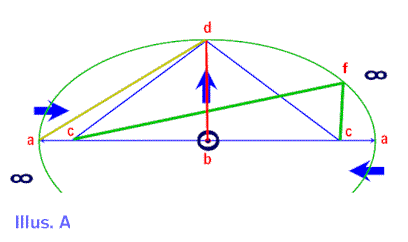In Summation: The significance of all numbers of the Brunardot Series and the Tinicir Series being Natural Integers is that they represent a soliton or a multiple of a soliton. Partial solitons do not exist anymore than partial waves or partial "charges" exist. The underlying principles of the most complex, by definition, must be the most simple. Mathematics originates with Natural phenomena; therefore, the simplest mathematics must reflect the most underlying principles of the most complex Natural phenomena. All the endless structures which result from the Brunardot Series and the Taicir Series are heuristic representations of three-dimensional energy pulses which are generated from and about axes that are infinitely long and infinitesimally narrow. As said pulses dissipate with the increase of time, to their originating singularity, their internal oscillations slow, they expand relativistically, and their dissipation is countered with increased pulses from along their locus of Reality, from which they originated. Thus, the Equilibrium Theory of  Reality with endless cycles of pulsating ellipsoids; their compression to atoms within quasars; and their dissipation as pulsating, elliptical strings. Conventional dark matter and dark energy are largely manifestations of said pulsating ellipsoids. Gravitation and Cosmic Inertia are largely a manifestation of said compression. Conventional Light, dissipents, is a manifestation of said dissipation.

 Summary: Seminal motion manifests as an unending, elliptical pulse, which is described by Brunardot Ellipses forming quaquametrically about an Infinity Line.  Said ellipses are defined by the Brunardot Series. Brunardot Ellipses define the shape of Reality, a singularity, as spherical; and, the shape of the Universe as elliptical. Brunardot Ellipses demonstrate, as the Brunardot Series reveals, that the Fibonacci series is incomplete because two of its most salient terms are not included . . . the very terms which establish its enigmatic character. Brunardot Harmonic Ellipses define the internal structure of mass and Light and the source and destination of their unending dissipation . . . and entanglement. The compression of Pulsoids, which are Brunardot Ellipsoids, and  its counter reaction manifests as gravitational "attraction" and Cosmic Inertia. Pulsoids are prototypical mass and Light; the source of  most "dark" matter and "dark" energy.  Pulsoids are analogous to Einstein's fields and the "fabric of space."  The Brunardot  Series is unique in that it defines certain classes of ellipses; all of which determine the Conceptual Unit . . ."One," 1.  The Conceptual Unit is referred to as "the Proof of One."The Brunardot Series, additionally, locks together the Golden Ratio, Phi, F; Pi, p, the ratio of the circle; and, completes the Fibonacci series as the second sequence of the Brunardot Series.

 E-mail :  Brunardot@Brunardot.com
 There is one Universe.
 It is perpetual, in equilibrium;
 and, a manifestation of the Unified Concept; thus; . . . the Fundamental Postulate.
 also,
 are a single discipline, Philogic, which proclaims perpetuity
 and the nexus of Life; such is
 . . . Conceptualism.This symbol indicates: not complete, or more information needed . . . please E-mail your information, corrections, documents, photos, inquiries, or any other needs to: Brunardot@Brunardot.com Return to Top of PageBrunardot.com websiteDedicated to Giordano Bruno, Leonardo da Vinci. Leonardo da Pisa, Arturo Meniot, & Denis Diderot Terms of:  © Copyright 1999-2017 by BrunardotCONCEPTUAL Applications Family of Informational Websites  Emphasizing Philogic, a discipline that overarches Science, Theology, and Philosophy (STP) Website Design by:   CONCEPTUAL  Applications a division of: WebComm21